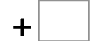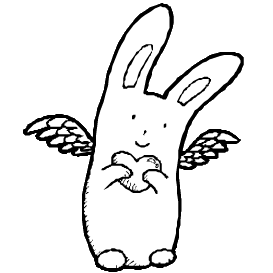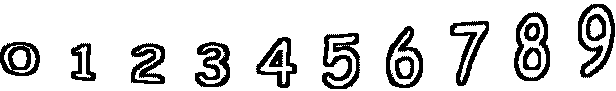31= 70   10= 6616= 33   23= 43   51= 119   59= 150 39= 97   74= 100   46= 131   48= 99